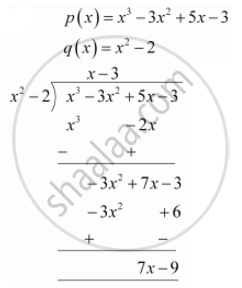Share
Notifications

View all notifications
Books Shortlist
Your shortlist is empty

# Divide the polynomial p(x) by the polynomial g(x) and find the quotient and remainder in each of the following p(x) = x^3 – 3x^2 + 5x – 3, g(x) = x2 – 2 - CBSE Class 10 - Mathematics

Login
Create free account

Forgot password?
ConceptDivision Algorithm for Polynomials

#### Question

Divide the polynomial p(x) by the polynomial g(x) and find the quotient and remainder in each of the following

p(x) = x3 – 3x2 + 5x – 3, g(x) = x2 – 2

#### SolutionQuotient = x - 3

Remainder = 7x - 9

Is there an error in this question or solution?

#### APPEARS IN

NCERT Solution for Mathematics Textbook for Class 10 (2019 to Current)
Chapter 2: Polynomials
Ex.2.30 | Q: 1.1 | Page no. 36

#### Video TutorialsVIEW ALL 

Solution Divide the polynomial p(x) by the polynomial g(x) and find the quotient and remainder in each of the following p(x) = x^3 – 3x^2 + 5x – 3, g(x) = x2 – 2 Concept: Division Algorithm for Polynomials.
S